#Function Repository Resource:

# SqrtSpace

Move back and forth from the squared space or square root space of an algebraic number field

Contributed by: Ed Pegg Jr
 ResourceFunction["SqrtSpace"][root,pts] while tracking signs, converts Cartesian pts2 to algebraic values in ℚ(root) or converts those values back to Cartesian.

## Examples

### Basic Examples

Using ϕ, the golden ratio or Fibonacci’s rabbit constant, convert points to the algebraic number field (ϕ) and build the Fermat triangle:

 In:=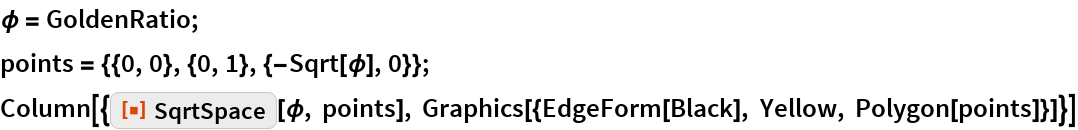Out=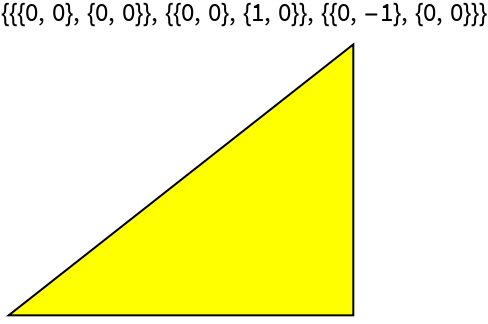Using ψ, the supergolden ratio or Narayana’s cow constant, convert points to the algebraic number field (ψ) and build the supergolden triangle:

 In:=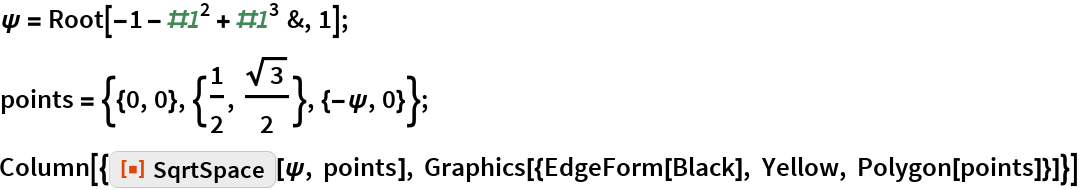Out=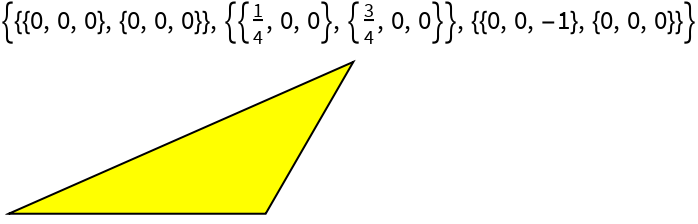Convert back to the original points:

 In:=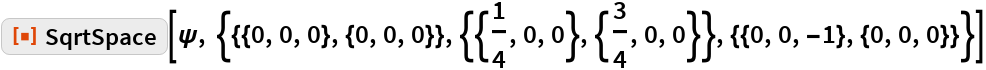Out=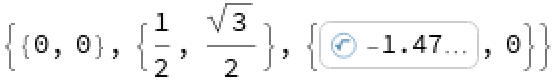### Properties and Relations

Under “Neat Examples” in GeometricScene, there is a mysterious output after “Decompose a triangle into similar triangles”:

 In:=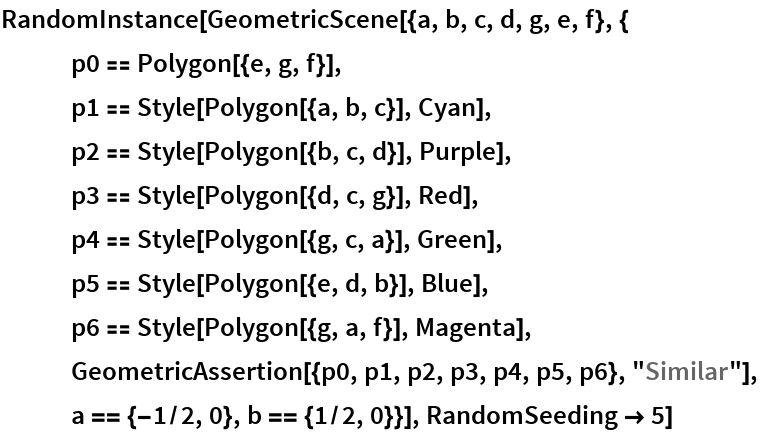Out=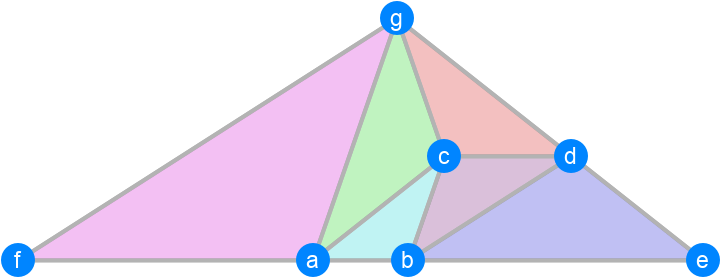This triangle is in the algebraic number field / geometric space of (ρ) where ρ is the plastic constant:

 In:=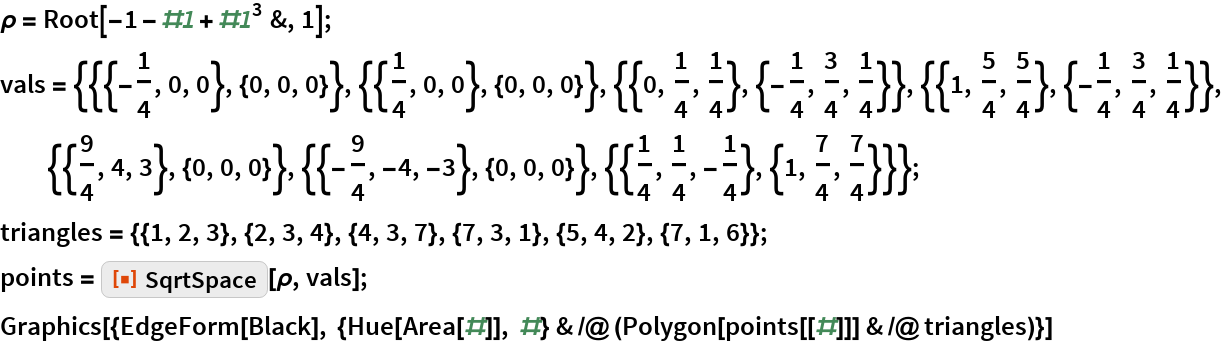Out=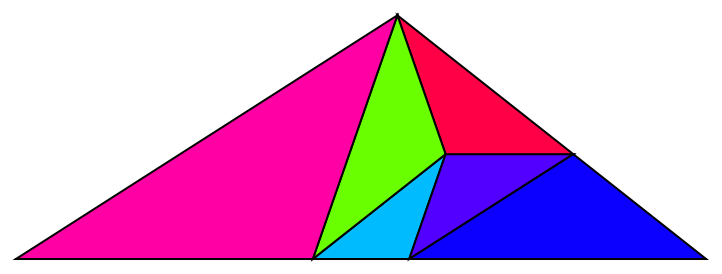Convert the points back to original values:

 In:=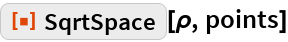Out=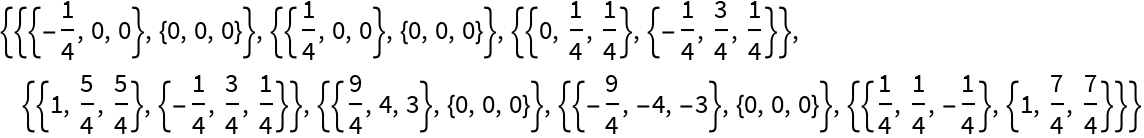A simple application of ToNumberField does not recognize the points as being in either (ρ) or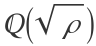, but does recognize the values when they get squared:

 In:=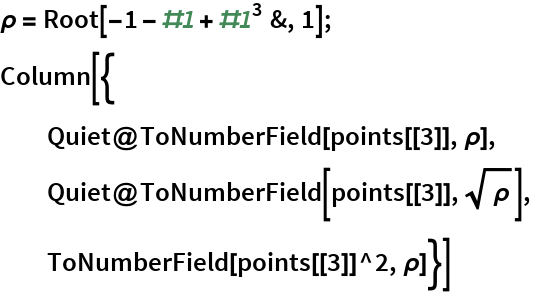Out=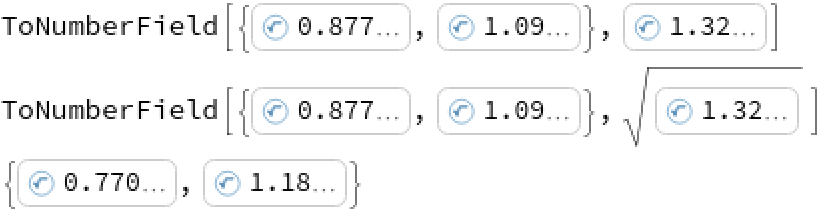These values are algebraic numbers:

 In:=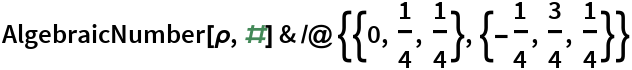Out=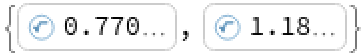The actual point is also a pair of algebraic numbers:

 In:=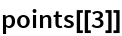Out=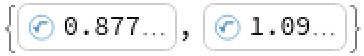The signs here happen to be positive, so taking the square root of the algebraic version does not require extra steps:

 In:=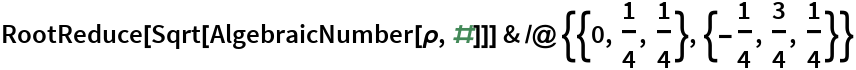Out=### Neat Examples

Convert 19 points from the algebraic number field (ρ) of the plastic constant ρ into 3D coordinates:

 In:=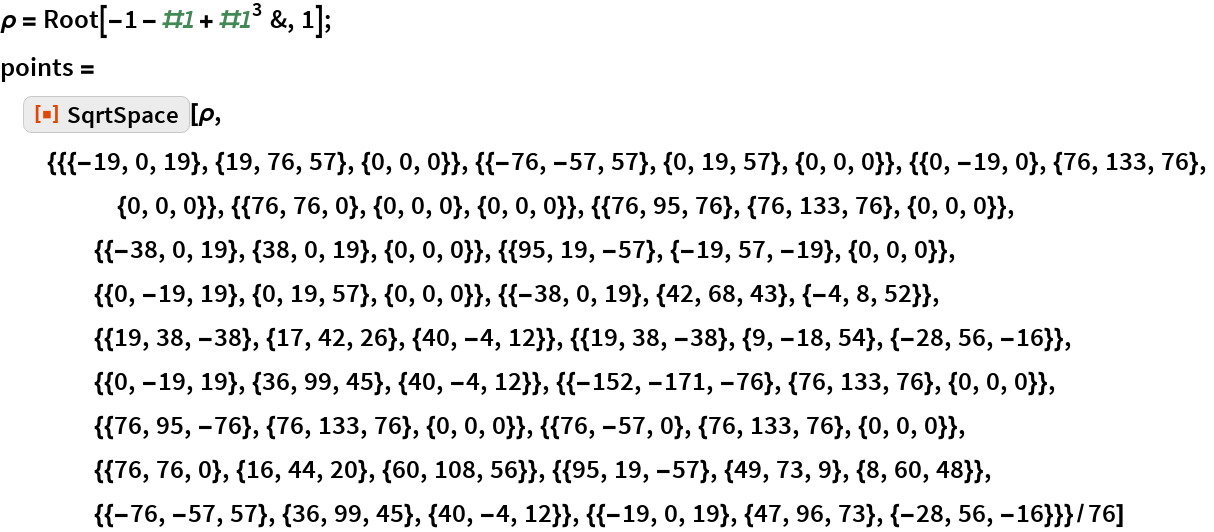Out=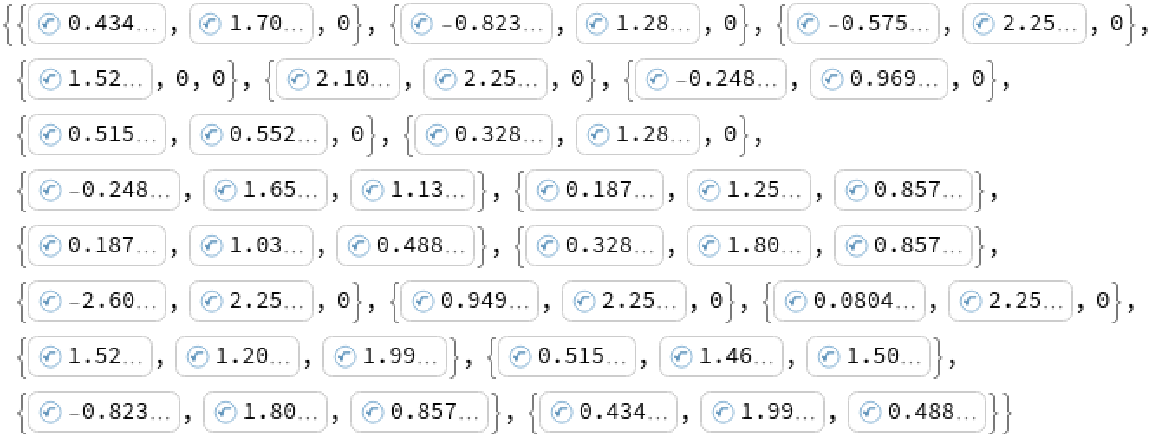Find the distances between these points in terms of powers of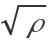:

 In:=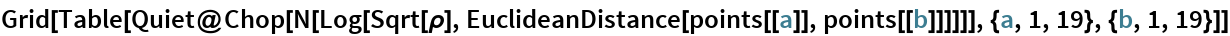Out=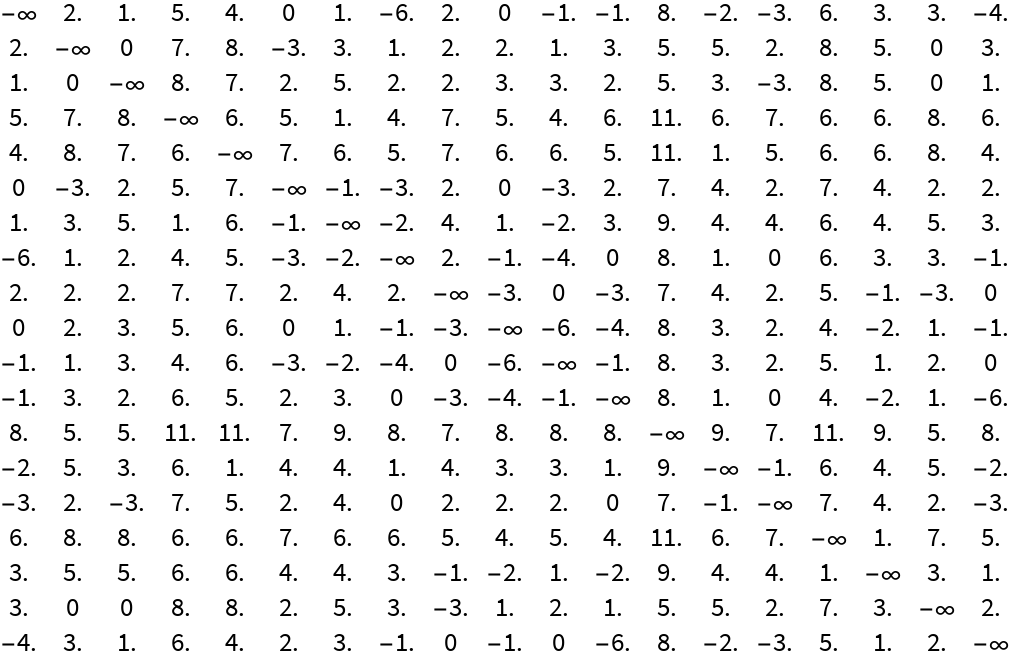Plot out the points:

 In:=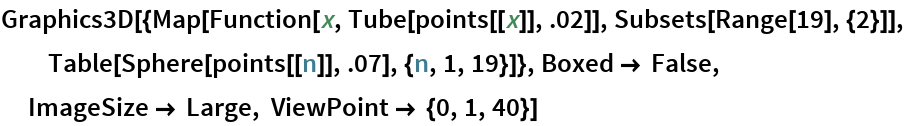Out=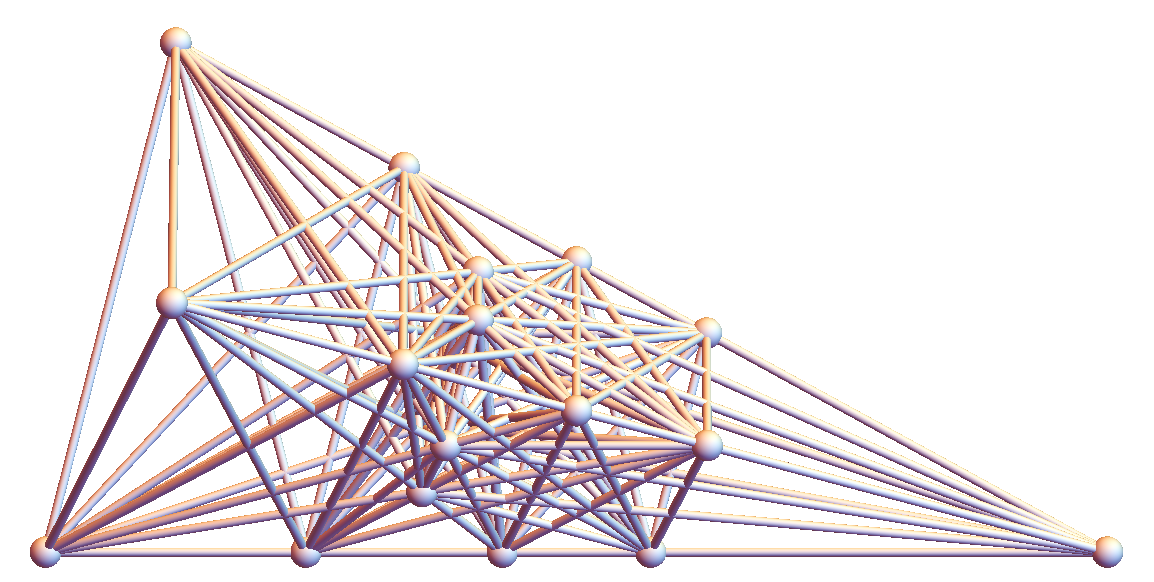## Requirements

Wolfram Language 11.3 (March 2018) or above